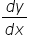Maths-
General
Easy

###Hint:

Slope of tangent =find equation of tangent at P, then find the intersection point of the tangent and curve by substituting the given values in the option, and whosoever satisfies the equation is the required point.

## Book A Free Demo+91